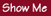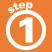Home    |    Teacher    |    Parents    |    Glossary    |    About UsWe use certain properties of integers to solve math problems:

1. Commutative property of addition2. Commutative property of multiplication3. Associative property of addition4. Associative property of muliplication5. Distributive propertyHomework Help | Pre-Algebra | NumbersEmail this page to a friendSearch·  Place value·  Decimal numbers·  Estimating and     rounding·  Adding / subtracting     decimals·  Multiplying decimals·  Dividing decimals·  Percent·  Exponents·  Square roots·  Signed integers·  Adding and     subtracting integers·  Multiplying and     dividing integers·  Properties of integersFirst Glance In Depth Examples WorkoutProperties of integers